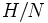# Prime power order implies no proper nontrivial characteristic direct factor

(diff) ← Older revision | Latest revision (diff) | Newer revision → (diff)

## Statement

Suppose$P$ is a group of prime power order. Then$P$ has no proper nontrivial characteristic direct factor. In other words, there is no proper nontrivial characteristic subgroup that is also a factor in a direct product.

## Facts used

1. Nilpotent implies every maximal subgroup is normal

## Proof

Given: A group$P$ of prime power order, expressed as an internal direct product of nontrivial subgroups$H$ and$K$.

To prove:$H$ is not characteristic in$P$.

Proof: Let$N$ be a maximal subgroup of$H$. Then,$N$ is normal and$H/N$ is cyclic of prime order. Let$Q$ be a subgroup of order$p$ contained in the center of$K$. Thus, we can construct a surjective homomorphism$\alpha:H \to Q$ with kernel$N$.

Now, consider the map:$\sigma: (h,k) \mapsto (h,\alpha(h)k)$.

This map is clearly a bijection from$P$ to$P$. It is a homomorphism because$\alpha(h) \in Z(K)$. Thus,$\sigma$ is an automorphism of$P$. Moreover,$\sigma$ does not leave$H$ invariant, showing that$H$ is not characteristic.